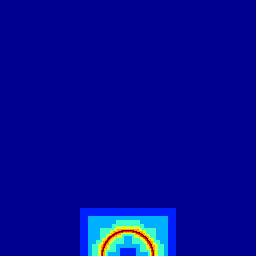# Check that user flags are properly reset when adapting

#include "fractions.h"
#include "utils.h"

#define LEVEL 12

static bool check_flags()
{
foreach_cell()
foreach_neighbor()
if (allocated(0))
for (int i = user; i <= user + 7; i++)
assert (!(cell.flags & (1 << i)));
return true;
}

int  main(int argc, char const *argv[])
{
size (10);
origin (-L0/2., 0.);
init_grid (256);

Initial refinement.

  scalar f[];
int iteration = 0;
do {
fraction (f, sq(x) + sq(y) - sq(1.));
}
while (check_flags() &&
adapt_wavelet ({f}, (double[]){1e-3}, LEVEL).nf != 0 &&
iteration++ <= 10);

for (int i = 0; i <= 5; i++) {
scalar s[];
foreach()
s[] = noise();

We adapt noise with zero tolerance i.e. the mesh should be refined everywhere down to LEVEl. The bug was triggered when minlevel is set.

    check_flags();
adapt_wavelet ({s}, (double []){0}, maxlevel = LEVEL, minlevel = 5);

This should eventually give a uniform refinement.Refinement levels

    scalar l[];
foreach()
l[] = level;
output_ppm (l, min = 5, max = LEVEL, file = "grid.gif");
}
}